Best for live in-class or video conferencing lessonsStart teacher-led lesson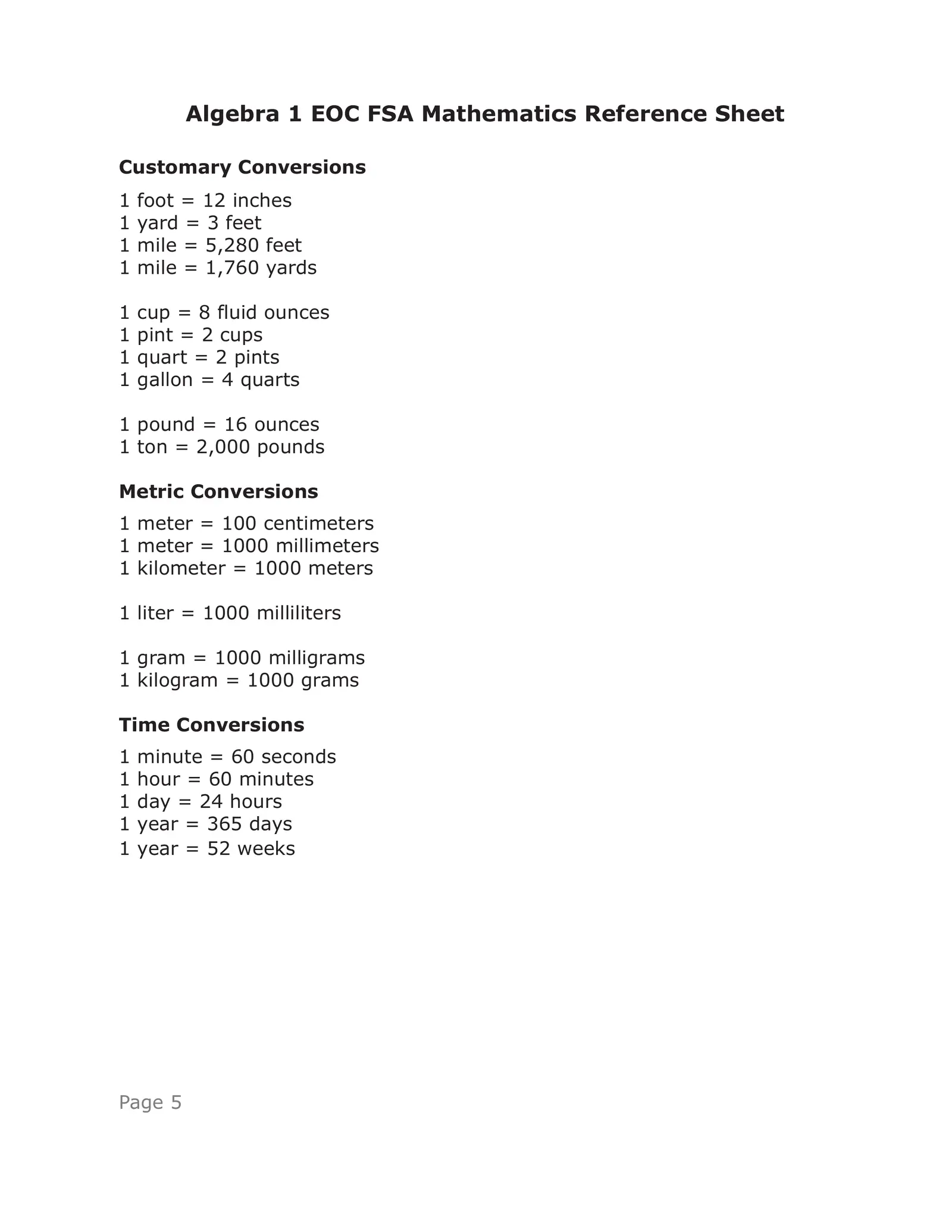Algebra 1 EOC FSA Mathematics Reference Sheet Customary Conversions 1 foot = 12 inches 1 yard = 3 feet 1 mile = 5,280 feet 1 mile = 1,760 yards 1 cup = 8 fluid ounces 1 pint = 2 cups 1 quart = 2 pints 1 gallon = 4 quarts 1 pound = 16 ounces 1 ton = 2,000 pounds Metric Conversions 1 meter = 100 centimeters 1 meter = 1000 millimeters 1 kilometer = 1000 meters 1 liter = 1000 milliliters 1 gram = 1000 milligrams 1 kilogram = 1000 grams Time Conversions 1 minute = 60 seconds 1 hour = 60 minutes 1 day = 24 hours 1 year = 365 days 1 year = 52 weeks Page 5BLANK PAGE Page 6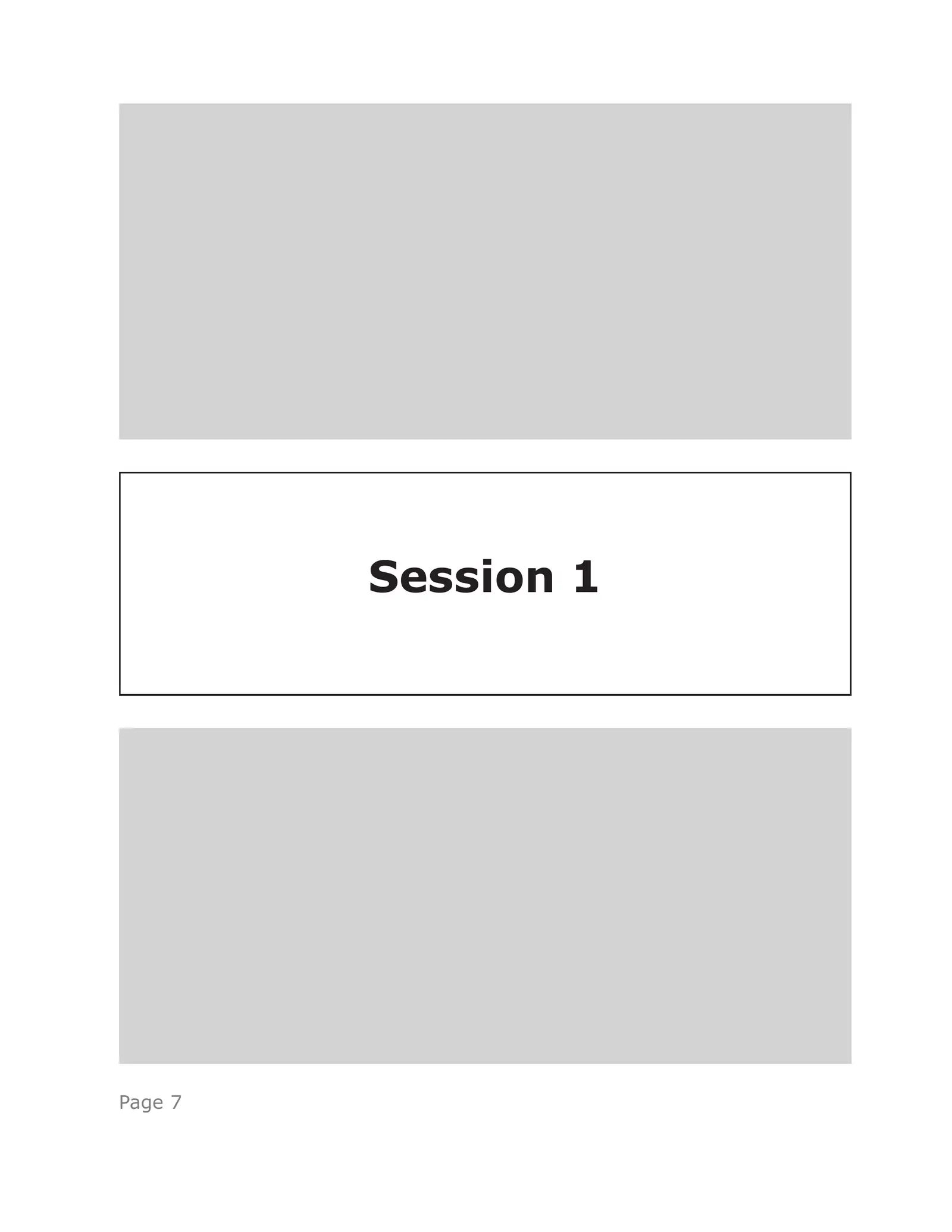Session 1 Page 7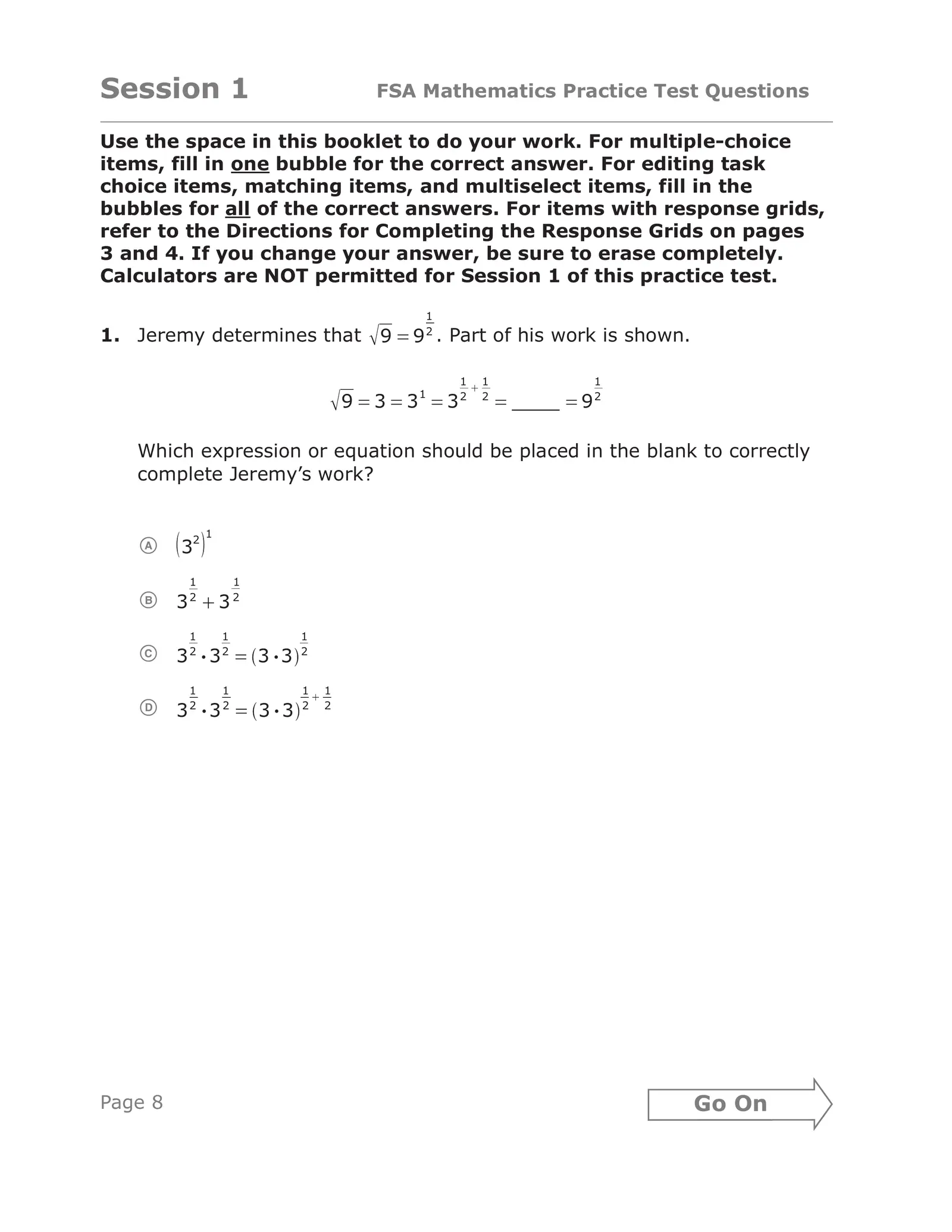Session 1 FSA Mathematics Practice Test Questions Use the space in this booklet to do your work. For multiple-choice items, fill in one bubble for the correct answer. For editing task choice items, matching items, and multiselect items, fill in the bubbles for all of the correct answers. For items with response grids, refer to the Directions for Completing the Response Grids on pages 3 and 4. If you change your answer, be sure to erase completely. Calculators are NOT permitted for Session 1 of this practice test. 1 1. Jeremy determines that 9 9 . Part of his work is shown. 2 1 1 1 1 2 2 2 9 3 3 3 9 Which expression or equation should be placed in the blank to correctly complete Jeremy’s work? 1 2 A 3 1 1 B 32 32 1 1 1 C 3 32 • 2 3 •3 2 1 1 1 1 D 3 32 • 2 3 3 • 2 2 15012 Page 8 Go On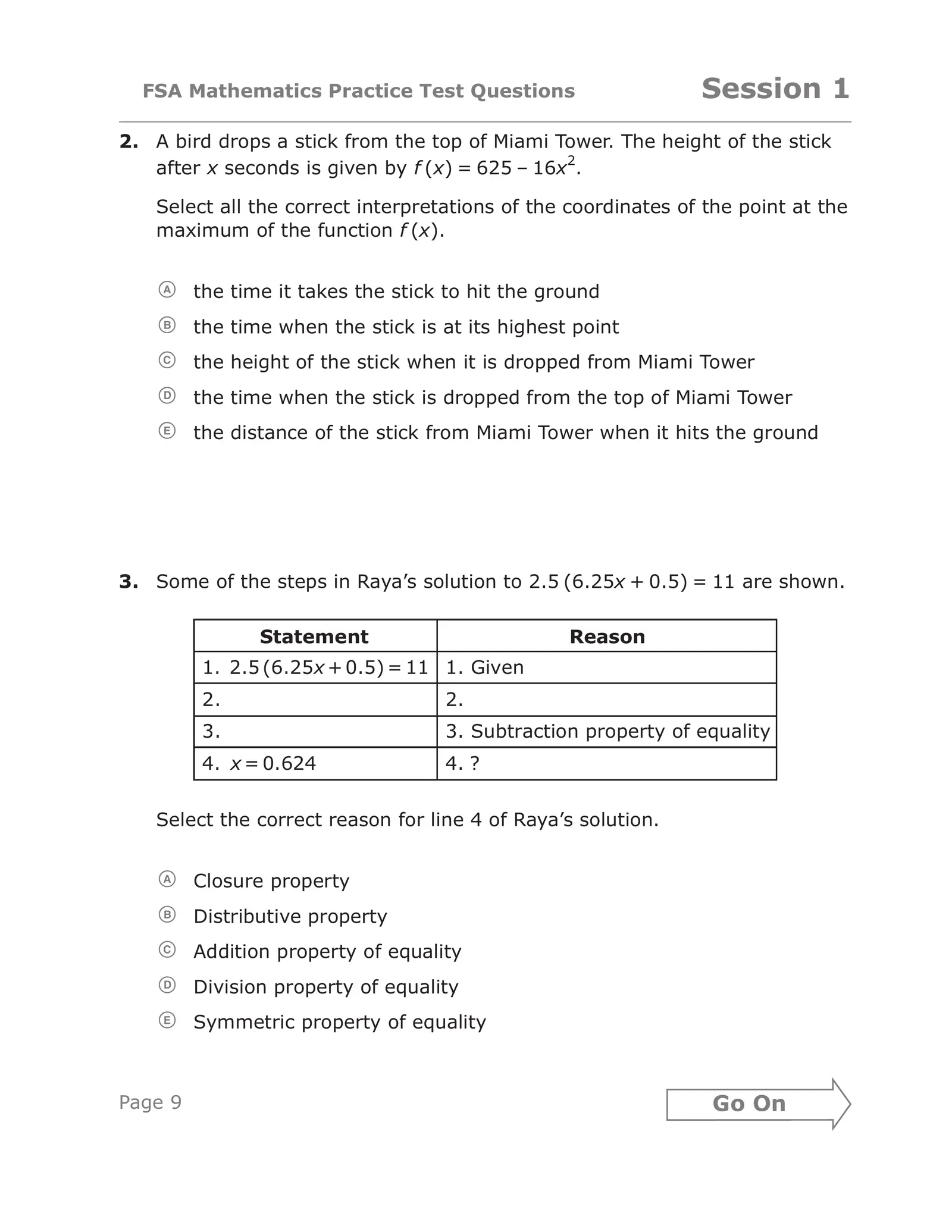FSA Mathematics Practice Test Questions Session 1 2. A bird drops a stick from the top of Miami Tower. The height of the stick 2 after x seconds is given by f (x) = 625 – 16x . 1010 Select all the correct interpretations of the coordinates of the point at the maximum of the function f (x). A the time it takes the stick to hit the ground B the time when the stick is at its highest point C the height of the stick when it is dropped from Miami Tower D the time when the stick is dropped from the top of Miami Tower E the distance of the stick from Miami Tower when it hits the ground 14706 3. Some of the steps in Raya’s solution to 2.5 (6.25x + 0.5) = 11 are shown. Statement Reason 1. 2.5 (6.25x + 0.5) = 11 1. Given 2. 2. 3. 3. Subtraction property of equality 4. x = 0.624 4. ? Select the correct reason for line 4 of Raya’s solution. A Closure property B Distributive property C Addition property of equality D Division property of equality E Symmetric property of equality 14592 Page 9 Go On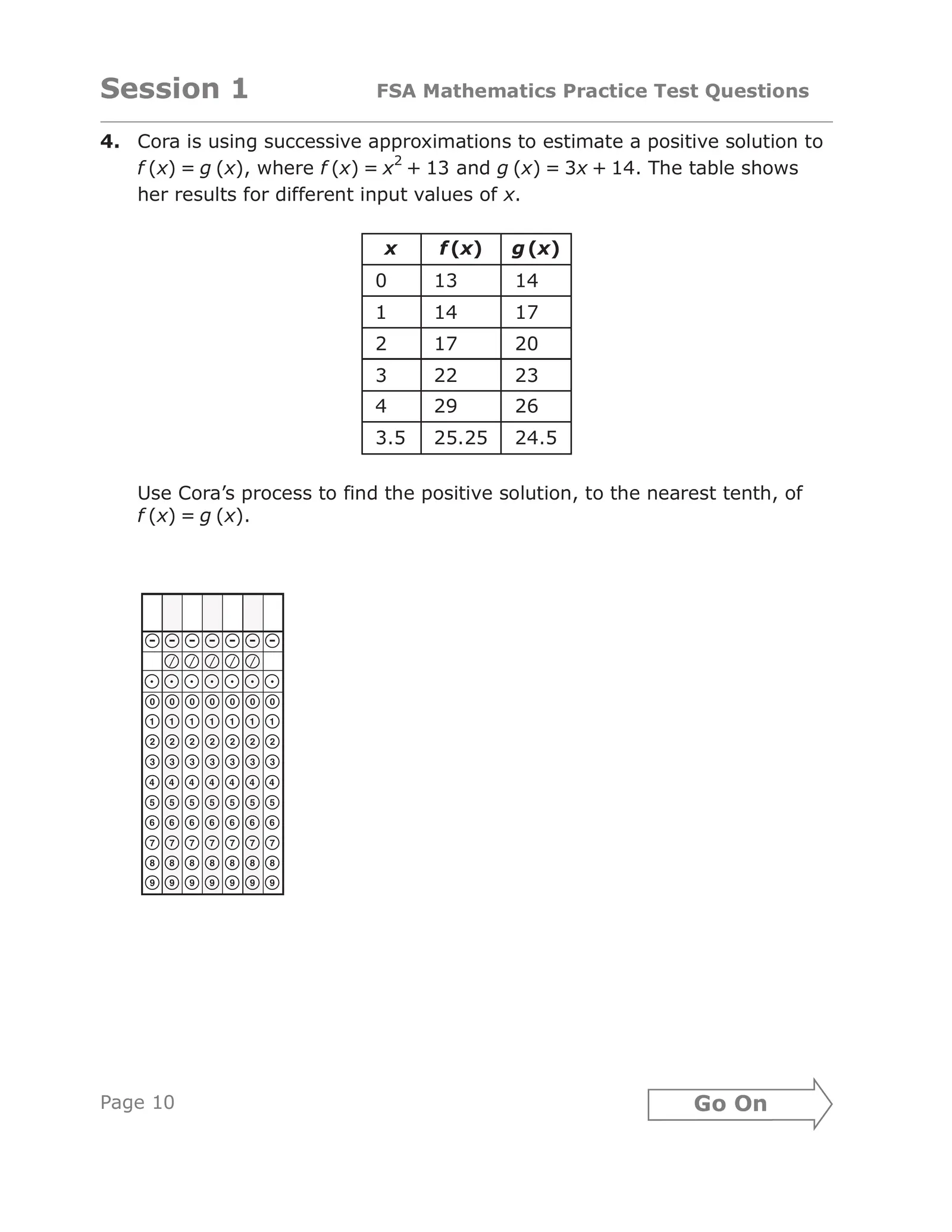Session 1 FSA Mathematics Practice Test Questions 4. Cora is using successive approximations to estimate a positive solution to 2 f (x) = g (x), where f (x) = x + 13 and g (x) = 3x + 14. The table shows her results for different input values of x. x f (x) g (x) 0 13 14 1 14 17 2 17 20 3 22 23 4 29 26 3.5 25.25 24.5 Use Cora’s process to find the positive solution, to the nearest tenth, of f (x) = g (x). ------- ````` aaaaaaa 0000000 1111111 2222222 3333333 4444444 5555555 6666666 7777777 8888888 9999999 14595 Page 10 Go On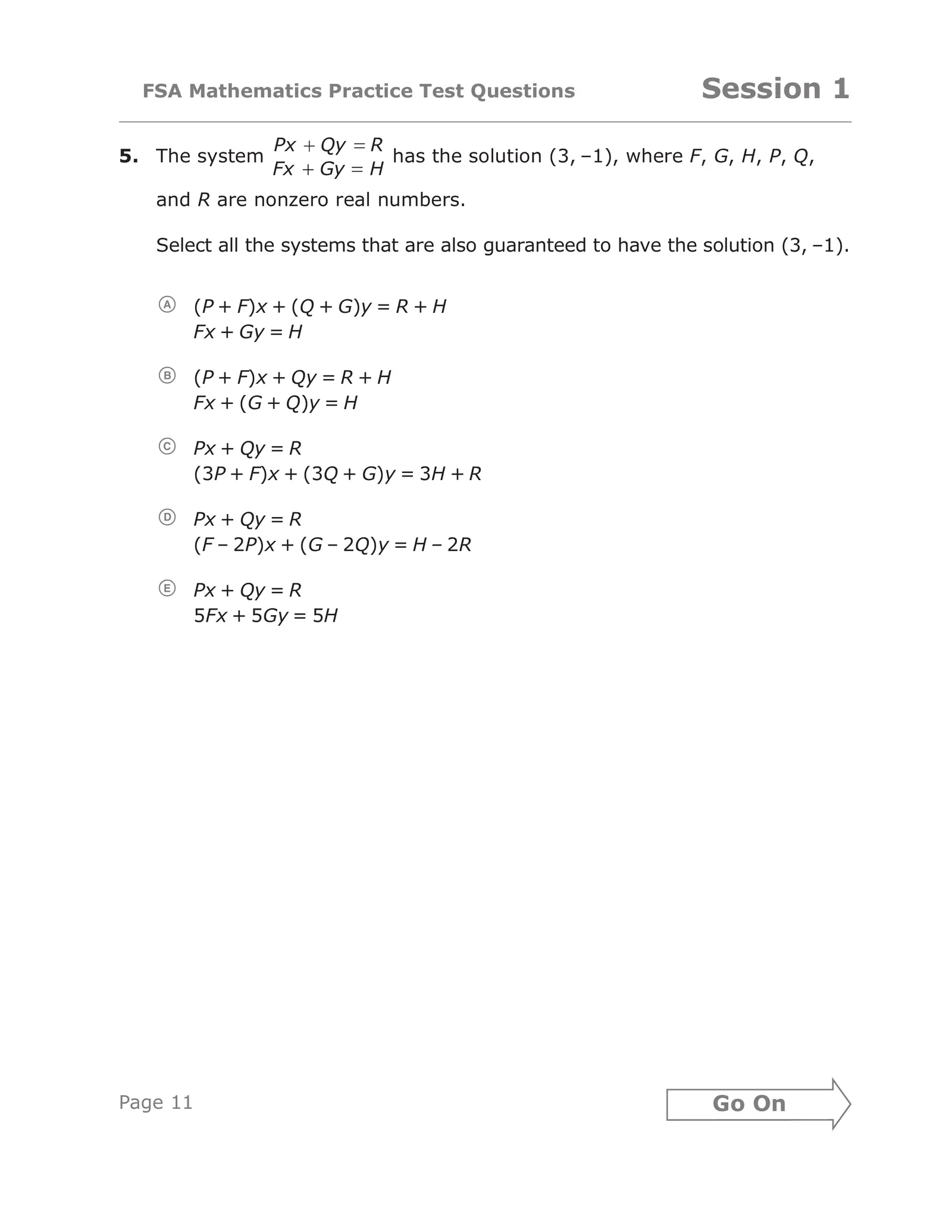FSA Mathematics Practice Test Questions Session 1 Px Qy R 5. The system has the solution (3, –1), where F, G, H, P, Q, Fx Gy H and R are nonzero real numbers. Select all the systems that are also guaranteed to have the solution (3, –1). A (P + F)x + (Q + G)y = R + H Fx + Gy = H B (P + F)x + Qy = R + H Fx + (G + Q)y = H C Px + Qy = R (3P + F)x + (3Q + G)y = 3H + R D Px + Qy = R (F – 2P)x + (G – 2Q)y = H – 2R E Px + Qy = R 5Fx + 5Gy = 5H 14581 Page 11 Go On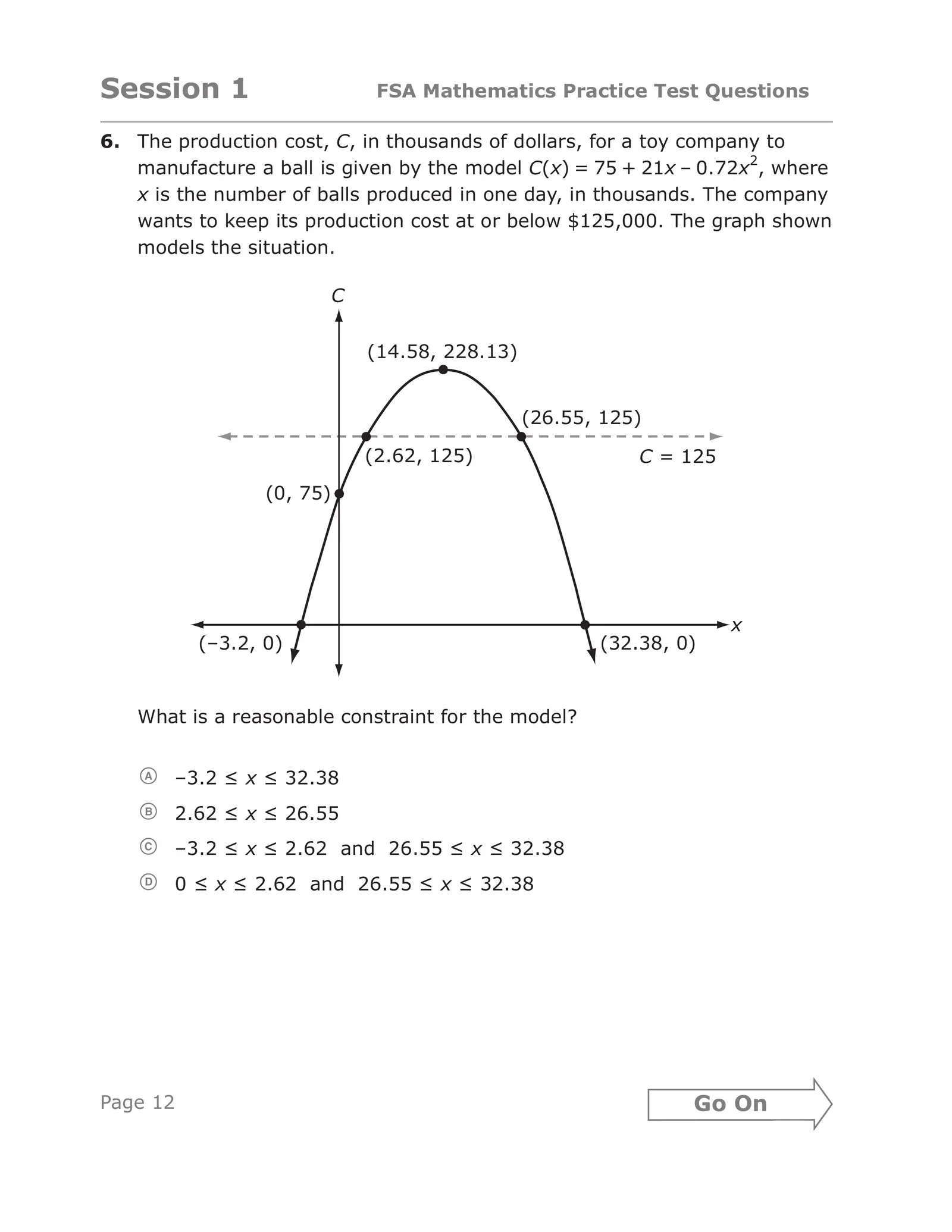Session 1 FSA Mathematics Practice Test Questions 6. The production cost, C, in thousands of dollars, for a toy company to manufacture a ball is given by the model C(x) = 75 + 21x – 0.72x2, where x is the number of balls produced in one day, in thousands. The company wants to keep its production cost at or below \$125,000. The graph shown models the situation. C (14.58, 228.13) (26.55, 125) (2.62, 125) C = 125 (0, 75) x (–3.2, 0) (32.38, 0) What is a reasonable constraint for the model? A –3.2 ≤ x ≤ 32.38 B 2.62 ≤ x ≤ 26.55 C –3.2 ≤ x ≤ 2.62 and 26.55 ≤ x ≤ 32.38 D 0 ≤ x ≤ 2.62 and 26.55 ≤ x ≤ 32.38 14582 Page 12 Go On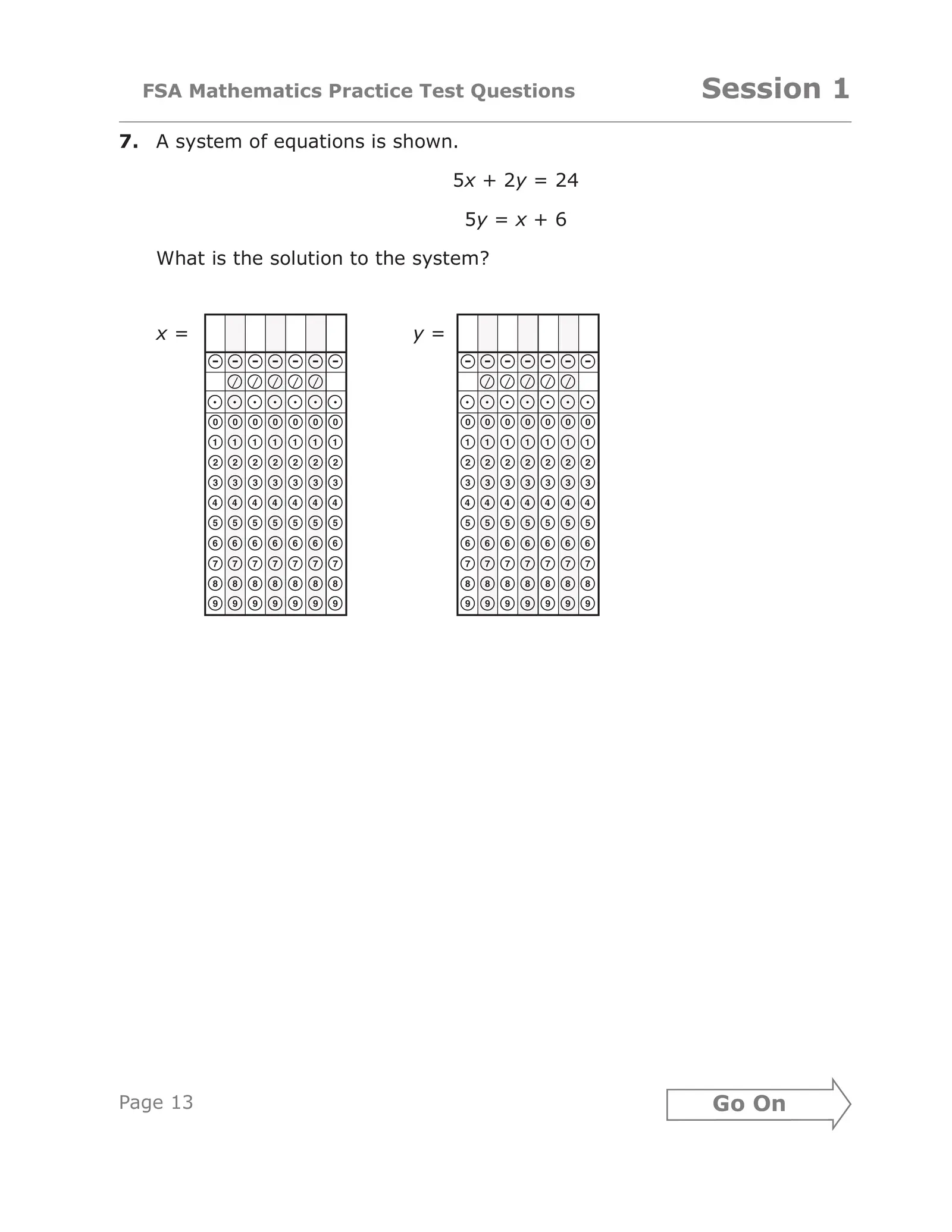FSA Mathematics Practice Test Questions Session 1 7. A system of equations is shown. 5x + 2y = 24 5y = x + 6 What is the solution to the system? x= y= ------- ------- ````` ````` aaaaaaa aaaaaaa 0000000 0000000 1111111 1111111 2222222 2222222 3333333 3333333 4444444 4444444 5555555 5555555 6666666 6666666 7777777 7777777 8888888 8888888 9999999 9999999 15236 Page 13 Go On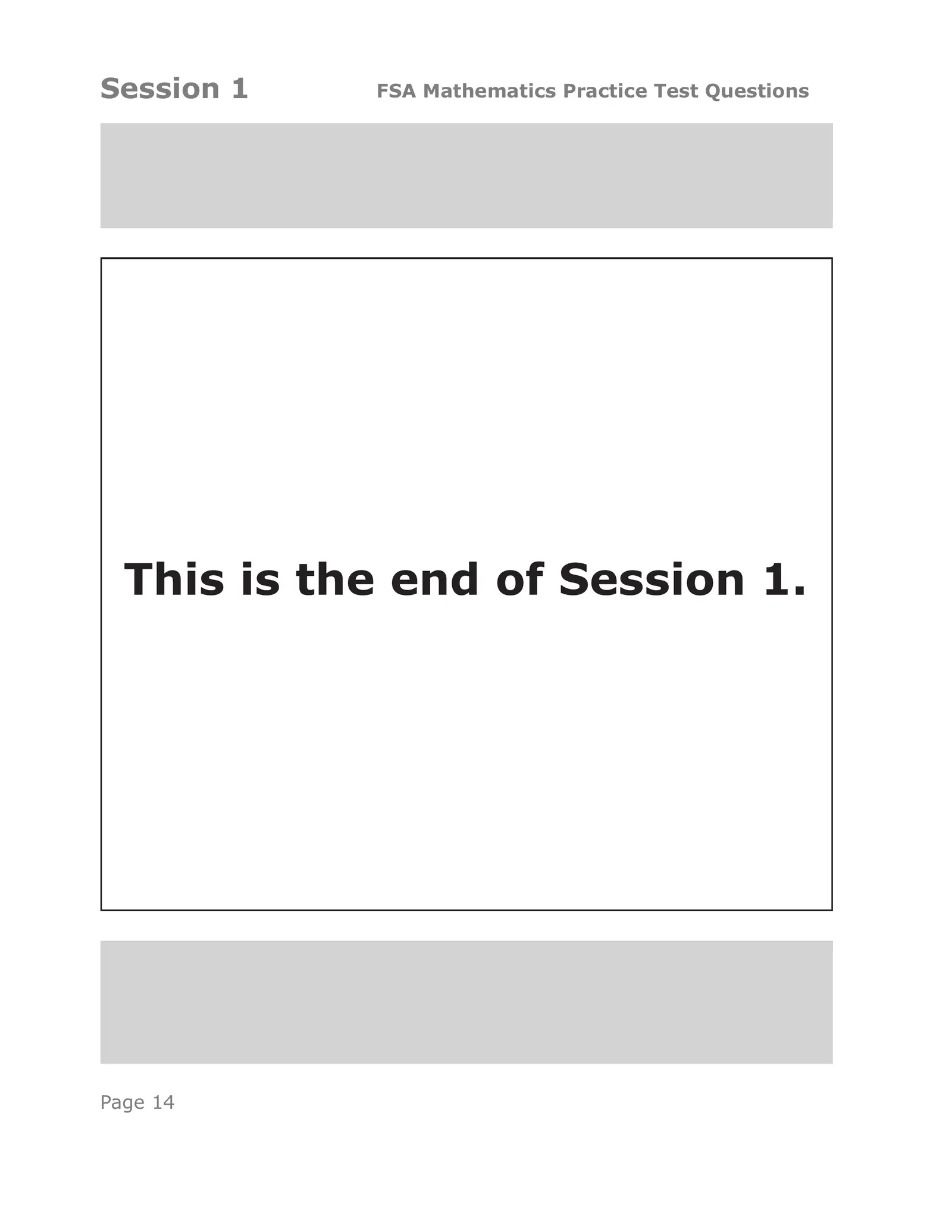Session 1 FSA Mathematics Practice Test Questions This is the end of Session 1. Page 14